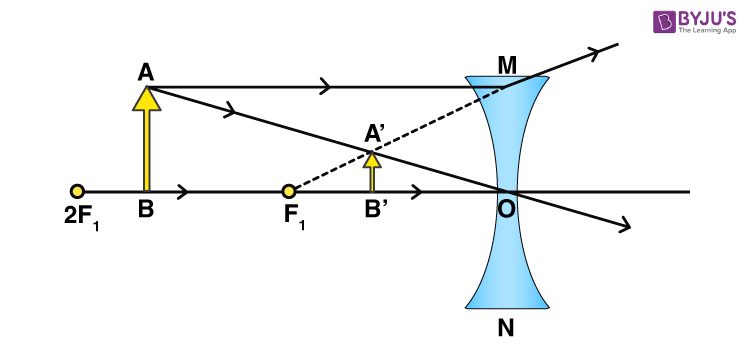# A concave lens of focal length 15 cm forms an image 10 cm from the lens. How far is the object placed from the lens? Draw the ray diagram.

Focal length (f ) = -15 cm

Distance of image (v) = -10 cm

$$\begin{array}{l}\frac{1}{v} – \frac{1}{u} = \frac{1}{f}\end{array}$$

1/u = -(1/10) – (1/- 15)

1/u =1/15 – 1/10

1/u = -0.033

u = -30 cm

So, the object is placed 30 cm away from the concave lens.

Ray diagram:(993)(200)